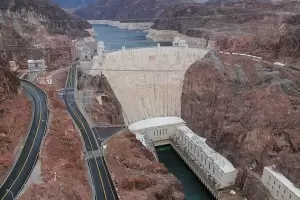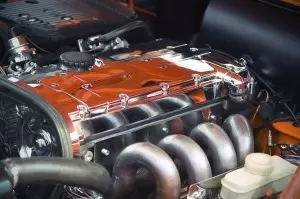# Mechanical Energy Examples in Everyday LifeMechanical energy is the energy that has the ability to do work. It is the sum of the kinetic energy and the potential energy of an object. According to the principle of conservation of energy, the sum of both forms of energy remains constant.

Kinetic energy depends on speed; it is the energy of motion. On the other hand, we can consider the potential energy as energy stored. This stored mechanical energy depends on:

• gravity (gravitational potential energy)

• or the elasticity of a material (elastic potential energy), for example, the potential energy stored in a spring.

The unit of mechanical energy in the international system is the joule (J)

Daily there are numerous examples of mechanical energy that help us to better understand this concept:

## 1. Throwing a Ball into the Air

Another example that illustrates how mechanical energy is the ability of an object to do work can be seen throwing a ball. It is an example of how the energy conversion principle works. During the launch, we distinguish the two types of mechanical energy:

1. The moment the ball leaves our hand with a certain speed, it has kinetic energy and no gravitational potential energy.

2. When the ball is at the highest point, its speed is 0, therefore, it has no kinetic energy. On the other hand, the ball has gained height. Therefore it does have potential energy.

During the process of going from point one to point two, all the kinetic energy has been converted into potential energy. As the ball falls, both types of energy will be reversed again.

## 2. Electric Motor

Motors have the ability to convert a specific type of energy into mechanical energy. An electric motor, in particular, transforms electricity into mechanical energy. Generally, the resulting energy is obtained in the form of rotational kinetic energy, an axis that rotates at a certain speed.

Daily we have many examples of electric motors:

• An electric washing machine.

• An electric scooter is a machine that uses mechanical energy to do work.

## 3. Electric Generator

An electric generator is a device that works the other way around than an electric motor. In this case, mechanical energy is made over into electrical power.

This conversion is achieved thanks to a magnetic field on the electrical conductors. When relative motion occurs between the conductors and the field, an electromotive force (EMF) will be generated.

This system is based on Faraday's law.

## 4. Hydropower PlantA hydropower plant is a way of transforming hydropower into electricity. It is a type of renewable energy. It uses the principle of energy conservation by taking advantage of the potential energy of the water stored in a reservoir.

These types of power plants accumulate water at a certain height. When they need to generate the energy, they drop it. At this time, they convert this energy into kinetic energy.

At the bottom of the plant, the water flow drives hydraulic turbines. The action of the turbines converts this energy into electrical energy using an electric generator and produces electricity.

## 5. Windmills

Wind energy is a form of energy capable of converting the force of the wind into mechanical energy. The energy associated with the wind can do work to the mill. Winds energy is the sum of all moving particles' kinetic energy.

When the wind hits the blades of a wind generator, the kinetic energy of each particle is transferred to the axis of the generator.

We can produce electricity using this mechanical energy. However, in the past, it was used directly in agriculture or industry.

## 6. Car's EngineThe engine of a gasoline car uses the chemical energy of fuel combustion to obtain thermal energy. The energy obtained in heat energy is used to drive a piston and generate cyclical linear motion (mechanical energy).

The cranks are in charge of converting this cyclical movement into a circular motion to rotate the wheels of the vehicle.

## 7.- Nuclear Energy

Nuclear power plants take advantage of the stored mechanical energy contained in the nucleus of an atom. When the atom is split after a fission reaction, part of its potential energy is converted into heat energy.

Heat energy is used to generate steam which its force acts against a turbine. The turbine has the ability to do work, and it is connected to an electrical generator that produces electricity.

Author:

Published: May 4, 2021
Last review: May 4, 2021